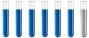## Electrochemistry question

Chemistry and homework help forum.

Organic Chemistry, Analytical Chemistry, Biochemistry, Physical Chemistry, Computational Chemistry, Theoretical Chemistry, High School Chemistry, Colledge Chemistry and University Chemistry Forum.

Share your chemistry ideas, discuss chemical problems, ask for help with scientific chemistry questions, inspire others by your chemistry vision!

Please feel free to start a scientific chemistry discussion here!

Discuss chemistry homework problems with experts!

Ask for help with chemical questions and help others with your chemistry knowledge!

Moderators: expert, ChenBeier, Xen

Dhamnekar Winod
Sr. Staff MemberPosts: 192
Joined: Sat Nov 21, 2020 10:14 am
Location: Mumbai[Bombay],Maharashtra State,India

### Electrochemistry question

A metal cup of surface area $$200. cm^2$$ needs to be electroplated with silver to a thickness of 0.200 mm. The density of silver is $$1.05 \times 10^4$$kg m⁻³.The mass of a silver ion is $$1 .79 \times$$ 10⁻²⁵ kg and the charge is the same magnitude as that on an electron. How long does the cup need to be in the electrolytic tank if a current of 12.5 A is being used?

Any chemistry help will be accepted. I am working on this question.
Any science consists of the following process. 1) See 2)Hear 3)Smell,if needed 4)Taste, if needed 5)Think 6)Understand 7)Inference 8)Take decision [Believe or disbelieve, useful or useless, true or false, cause or effect, any other criteria]
ChenBeier
Distinguished MemberPosts: 873
Joined: Wed Sep 27, 2017 7:25 am
Location: Berlin, Germany

### Re: Electrochemistry question

m = I*t*M(Ag)/(n*F)
m = Rho*V
V = th* A
Rho*th*A = I*t*M(Ag)/(n*F)
t= Rho*th*A* n*F/(I*M(Ag))

m= mass, I = current, t= time, M(Ag) molar mass of silver, n= amount of electrons to Transfer, F Faradyconstant, Rho = specific gravity of silver, th = thickness of plated silver, A = surface of cup
Dhamnekar Winod
Sr. Staff MemberPosts: 192
Joined: Sat Nov 21, 2020 10:14 am
Location: Mumbai[Bombay],Maharashtra State,India

### Re: Electrochemistry question

The volume of silver that gets electroplated is 0.00000400 m³.
The mass of silver that gets electroplated is 0.00000400 m³ × 1.05 × 10⁴ kg m⁻³=0.042 kg.

The molar mass of silver= 107.8682 g/mol , so moles of silver 42 g/107.87 g = 0.38935756 mole of silver.

Hence, moles of electrons required Ag ⁺ (aq) + e ⁺→ Ag(s), so 0.38935756 mole of silver requires 0.38935756 mole of electrons.

If i plugged in these values in your formula answer is $$t= \frac{42 g \times 0.38935756 mol e^- \times 96485 C/mol} {12. 5 C/ s \times 107.87 g} = 1170.165$$ seconds

But the correct answer given is 3005 seconds ~ 50 mins approx.

What is wrong here?
Any science consists of the following process. 1) See 2)Hear 3)Smell,if needed 4)Taste, if needed 5)Think 6)Understand 7)Inference 8)Take decision [Believe or disbelieve, useful or useless, true or false, cause or effect, any other criteria]
ChenBeier
Distinguished MemberPosts: 873
Joined: Wed Sep 27, 2017 7:25 am
Location: Berlin, Germany

### Re: Electrochemistry question

You devided two times by the molar mass.
If you calculated n = m/M then you cannot do the thing twices.
Dhamnekar Winod
Sr. Staff MemberPosts: 192
Joined: Sat Nov 21, 2020 10:14 am
Location: Mumbai[Bombay],Maharashtra State,India

### Re: Electrochemistry question

t= n*F/I , n= number of moles of electrons required, F= Faraday constant, I= current in Ampere or Coulombs/second.

t=(0.38935756 Moles* 96485 C)/12.5 C/s = 3005.37 seconds, or 50 minutes approx.
Any science consists of the following process. 1) See 2)Hear 3)Smell,if needed 4)Taste, if needed 5)Think 6)Understand 7)Inference 8)Take decision [Believe or disbelieve, useful or useless, true or false, cause or effect, any other criteria]
ChenBeier
Distinguished MemberPosts: 873
Joined: Wed Sep 27, 2017 7:25 am
Location: Berlin, Germany

### Re: Electrochemistry question

Yes, now its correct.